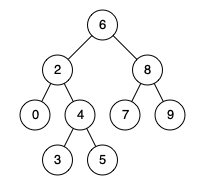# 235. Lowest common ancestor of a binary search tree

https://leetcode.com/problems/lowest-common-ancestor-of-a-binary-search-tree/

Given a binary search tree (BST), find the lowest common ancestor (LCA) of two given nodes in the BST. According to the definition of LCA on Wikipedia: “The lowest common ancestor is defined between two nodes p and q as the lowest node in T that has both p and q as descendants (where we allow a node to be a descendant of itself).”

Example 1:Input: root = [6,2,8,0,4,7,9,null,null,3,5], p = 2, q = 8 Output: 6 Explanation: The LCA of nodes 2 and 8 is 6. Example 2:Input: root = [6,2,8,0,4,7,9,null,null,3,5], p = 2, q = 4 Output: 2 Explanation: The LCA of nodes 2 and 4 is 2, since a node can be a descendant of itself according to the LCA definition.

• code
``````
class Solution {
public TreeNode lowestCommonAncestor(TreeNode root, TreeNode p, TreeNode q) {
if (root.val < q.val && root.val < p.val) return lowestCommonAncestor(root.right, p, q);
if (root.val > q.val && root.val > p.val) return lowestCommonAncestor(root.left, p, q);
return root;
}
}
``````
• code
``````class Solution:
def lowestCommonAncestor(self, root: 'TreeNode', p: 'TreeNode', q: 'TreeNode') -> 'TreeNode':
if p.val > q.val:
p, q = q, p
while root:
if root.val < p.val:
root = root.right
elif root.val > q.val:
root = root.left
# elif p.val < root.val < q.val or root.val == p.val or root.val == q.val:
else:
return root

``````